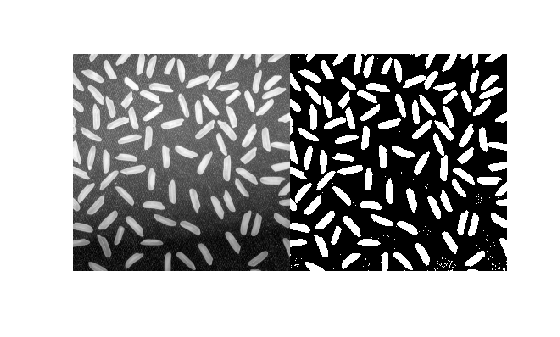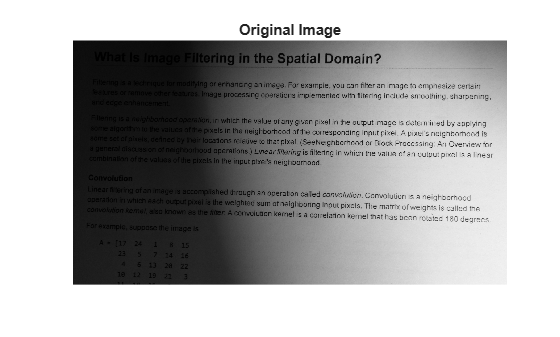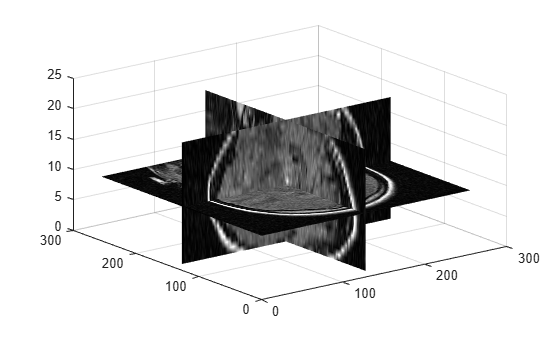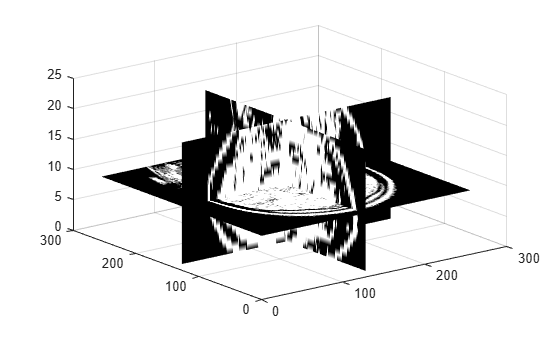# imbinarize

Binarize 2-D grayscale image or 3-D volume by thresholding

## Syntax

``BW = imbinarize(I)``
``BW = imbinarize(I,method)``
``BW = imbinarize(I,T)``
``BW = imbinarize(I,'adaptive',Name,Value)``

## Description

example

````BW = imbinarize(I)` creates a binary image from 2-D or 3-D grayscale image `I` by replacing all values above a globally determined threshold with `1`s and setting all other values to `0`s. By default, `imbinarize` uses Otsu's method, which chooses the threshold value to minimize the intraclass variance of the thresholded black and white pixels . `imbinarize` uses a 256-bin image histogram to compute Otsu's threshold. To use a different histogram, see `otsuthresh`.```

example

````BW = imbinarize(I,method)` creates a binary image from image `I` using the thresholding method specified by `method`: `'global'` or `'adaptive'`.```
````BW = imbinarize(I,T)` creates a binary image from image `I` using the threshold value `T`. `T` can be a global image threshold, specified as a scalar luminance value, or a locally adaptive threshold, specified as a matrix of luminance values.```

example

````BW = imbinarize(I,'adaptive',Name,Value)` creates a binary image from image `I` using name-value pairs to control aspects of adaptive thresholding.```

## Examples

collapse all

Read grayscale image into the workspace.

`I = imread('coins.png');`

Convert the image into a binary image.

`BW = imbinarize(I);`

Display the original image next to the binary version.

```figure imshowpair(I,BW,'montage')````I = imread('rice.png');`

Convert grayscale image to binary image.

`BW = imbinarize(I, 'adaptive');`

Display original image along side binary version.

```figure imshowpair(I,BW,'montage')```Read a grayscale image into the workspace and display it.

```I = imread('printedtext.png'); figure imshow(I) title('Original Image')```Convert the image to a binary image using adaptive thresholding. Use the `ForegroundPolarity` parameter to indicate that the foreground is darker than the background.

`BW = imbinarize(I,'adaptive','ForegroundPolarity','dark','Sensitivity',0.4);`

Display the binary version of the image.

```figure imshow(BW) title('Binary Version of Image')```Load 3-D grayscale intensity data into the workspace.

```load mristack; V = mristack;```

View the 3-D volume.

```figure slice(double(V),size(V,2)/2,size(V,1)/2,size(V,3)/2) colormap gray shading interp```Convert the intensity volume into a 3-D binary volume.

`J = imbinarize(V);`

View the 3-D binary volume.

```figure slice(double(J),size(J,2)/2,size(J,1)/2,size(J,3)/2) colormap gray shading interp```## Input Arguments

collapse all

Input image, specified as a 2-D grayscale image or a 3-D grayscale volume. `imbinarize` expects pixel values of data type `double` and `single` to be in the range [0, 1]. You can use the `rescale` function to adjust pixel values to the expected range.

Note

`imbinarize` interprets an RGB image as a volumetric grayscale image and does not binarize each channel separately. To produce a binary image from an RGB image, first convert the image to a grayscale image using `rgb2gray`.

Data Types: `single` | `double` | `int8` | `int16` | `int32` | `uint8` | `uint16` | `uint32`

Method used to binarize image, specified as one of the following values.

Values

Meaning

`'global'`

Calculate global image threshold using Otsu's method. See `graythresh` for more information about Otsu’s method.

`'adaptive'`

Calculate locally adaptive image threshold chosen using local first-order image statistics around each pixel. See `adaptthresh` for details. If the image contains `Inf`s or `NaN`s, the behavior of `imbinarize` for the `'adaptive'` method is undefined. Propagation of `Inf`s or `NaN`s might not be localized to the neighborhood around `Inf` and `NaN` pixels.

Data Types: `char` | `string`

Threshold luminance value, specified as a numeric scalar or numeric array with values in the range [0, 1].

• If `T` is a numeric scalar, then `imbinarize` interprets `T` as a global image threshold. Use `graythresh` or `otsuthresh` to compute a global image threshold.

• If `T` is a numeric array, then `imbinarize` interprets `T` as a locally adaptive threshold. Use `adaptthresh` to compute a locally adaptive threshold.

Data Types: `single` | `double` | `int8` | `int16` | `int32` | `int64` | `uint8` | `uint16` | `uint32` | `uint64`

### Name-Value Arguments

Specify optional comma-separated pairs of `Name,Value` arguments. `Name` is the argument name and `Value` is the corresponding value. `Name` must appear inside quotes. You can specify several name and value pair arguments in any order as `Name1,Value1,...,NameN,ValueN`.

Example: `BW = imbinarize(I,'adaptive','Sensitivity',0.4);`

Sensitivity factor for adaptive thresholding, specified as the comma-separated pair consisting of `'Sensitivity'` and a number in the range [0, 1]. A high sensitivity value leads to thresholding more pixels as foreground, at the risk of including some background pixels.

Data Types: `single` | `double` | `int8` | `int16` | `int32` | `int64` | `uint8` | `uint16` | `uint32` | `uint64`

Determine which pixels are considered foreground pixels for adaptive thresholding, specified as the comma-separated pair consisting of `'ForegroundPolarity'` and one of the following values.

Value

Meaning

`'bright'`

The foreground is brighter than the background.

`'dark'`

The foreground is darker than the background

Data Types: `char` | `string`

## Output Arguments

collapse all

Output binary image, returned as a logical matrix or logical array of the same size as `I`.

Data Types: `logical`

## Tips

• To produce a binary image from an indexed image, first convert the image to a grayscale image using `ind2gray`.

## Algorithms

The `'adaptive'` method binarizes the image using a locally adaptive threshold. `imbinarize` computes a threshold for each pixel using the local mean intensity around the neighborhood of the pixel. This technique is also called Bradley's method . The `'adaptive'` method also uses a neighborhood size of approximately 1/8th of the size of the image (computed as `2*floor(size(I)/16)+1`). To use a different first order local statistic or a different neighborhood size, see `adaptthresh`.

 Otsu, N., "A Threshold Selection Method from Gray-Level Histograms." IEEE Transactions on Systems, Man, and Cybernetics. Vol. 9, No. 1, 1979, pp. 62–66.

 Bradley, D., G. Roth, "Adapting Thresholding Using the Integral Image," Journal of Graphics Tools. Vol. 12, No. 2, 2007, pp.13–21.